# Box Plots

Using Analytica 5.2 or later versions, users can create box-whisker plots using the OnGraphDraw attribute and the set of relevant drawing functions. The following is an example of a box-whisker plot created using the new functionality: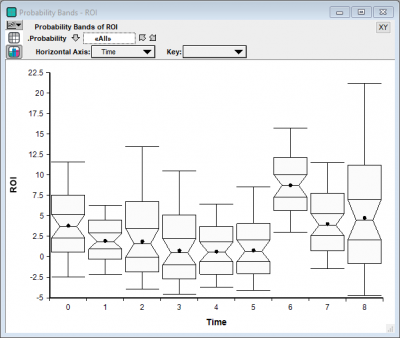Users of previous versions may use probability bands, as described below, to communicate similar information.

A classical box-whisker plot is one that looks like this: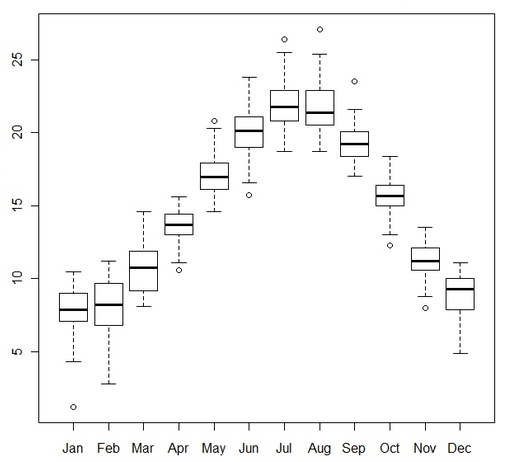The whisker-ends usually represent either the min and max range of data, or the 10% and 90% fractiles. The top and bottom of the box denotes the 25% and 75% quartiles, with the middle line being the median.

Analytica's probability bands graph is used to depict the same type information, but in a different format: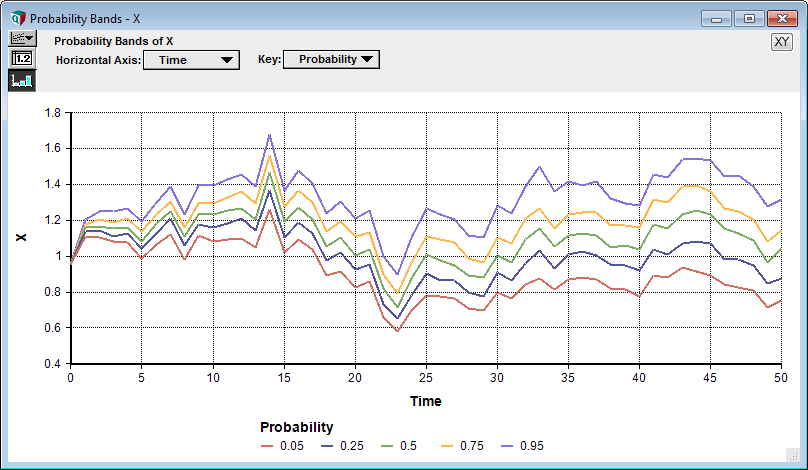As a substitute for a box-whisker plot, you can consider a "box-box-box plot":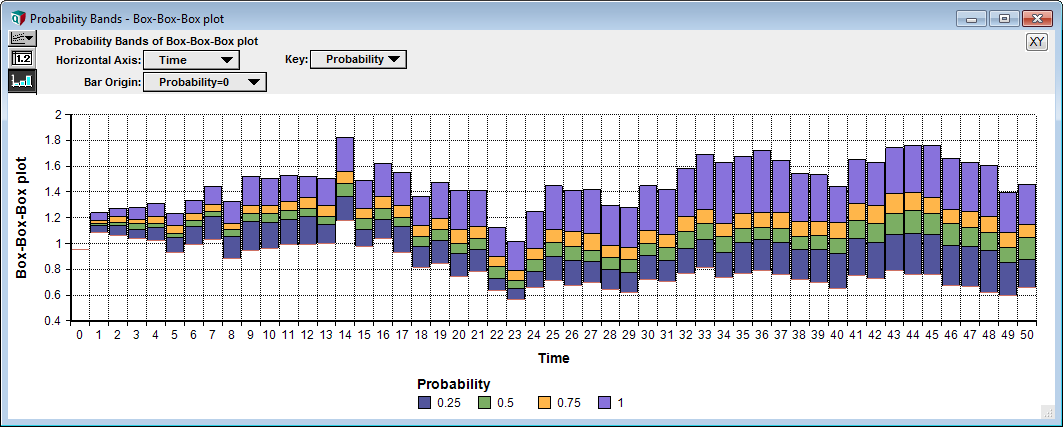Or, in the other direction, a whisker-whisker-whisker plot:While the topmost box-whisker plot is not currently available in Analytica 4.4, all three of the other variations are possible using Analytica's graphing engine. All three plots depict the same information.

## Probability Bands Plot

The probability bands plot is immediate in the result view when viewing an uncertain quantity that has at least one other dimension (usually a time dimension). Just select the ProbBands view in the top-left corner of the result view.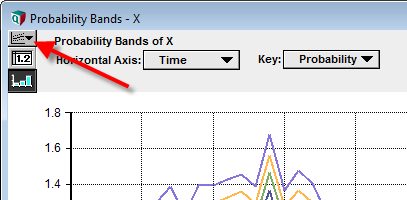## Box-Box-Box plots

To obtain a box-box-box plot, select a ProbBands result view, then double click to change the chart type settings. Select a bar chart, check the "Variable origin", and uncheck "Swap horizontal and vertical".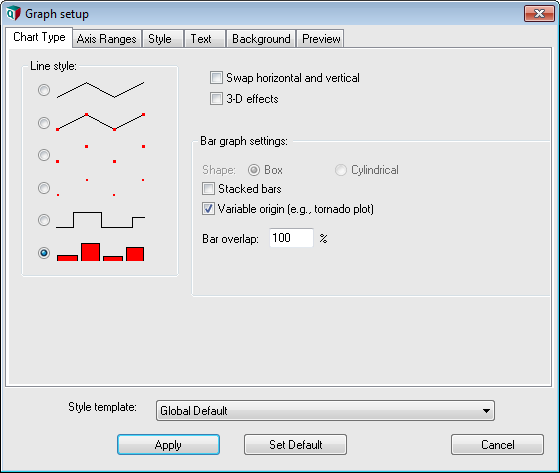Next, press Ctrl+U (Uncertainty Setup dialog) and select the probability levels of interest:Finally, ensure your horizontal axis is your time index, Key is Probability, and then for Bar Origin select Probability = 0 (or your lowest selected fractile):## Whisker-Whisker-Whisker plots

The Whisker-whisker-whisker plot is sometimes a little tricky to get right. Once again, display the ProbBands view. You must add your Time in as a comparison index, even though it already appears as a pivoter index. This enables the use of an XY graph with more flexible control over which series of points are connected by lines (the running index).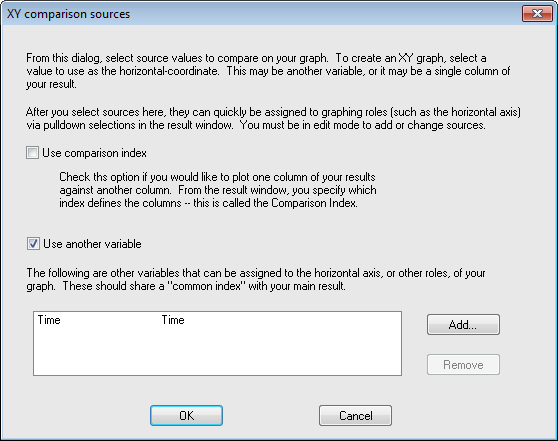You need to use a line-symbol chart type, and select Use Separate color / symbol keys:With this, you can now pivot as shown in the next final image to obtain the final graph: